# Practical Geometry Solutions Exercise 14.5 Class 6 Ncert

Ncert solutions for Mathematics Class 6th text book Chapter 14 Practical Geometry Exercise 14.5 are given.

Study the textbook lesson Practical Geometry very well.

Observe the example problems and solutions given in the textbook.

Observe the given below solutions and try them in your own method.

You can see Solutions for Class 6 Maths Ncert

Chapter 1 Knowing our Numbers

Chapter 2 whole Numbers

Some chapters solutions links are given below.

M

# Solutions for Practical Geometry Exercise 14.5 Ncert class 6th Maths

Maths Class 6 Chapter 14 Practical Geometry Exercise 14.5

Exercise 14.5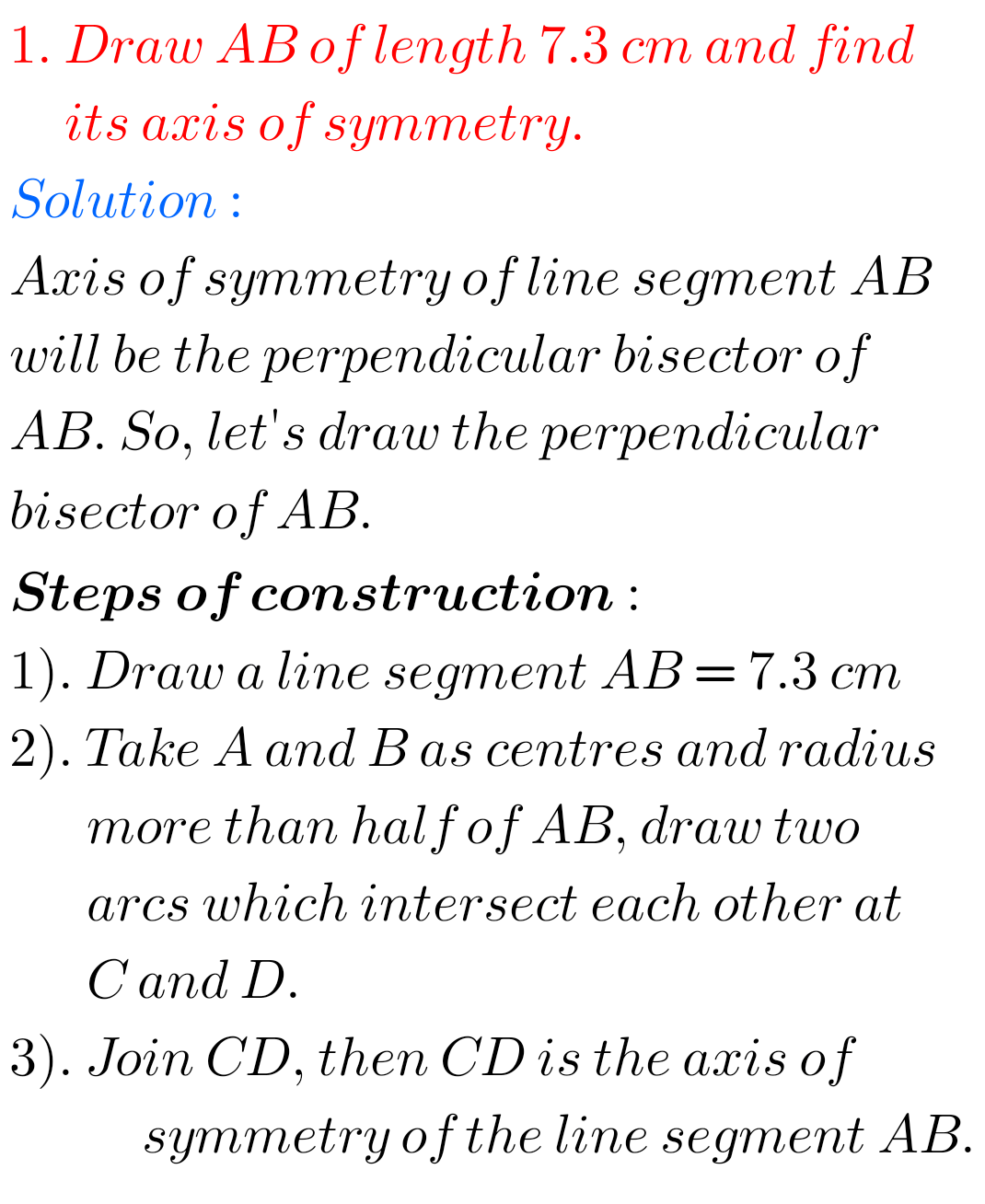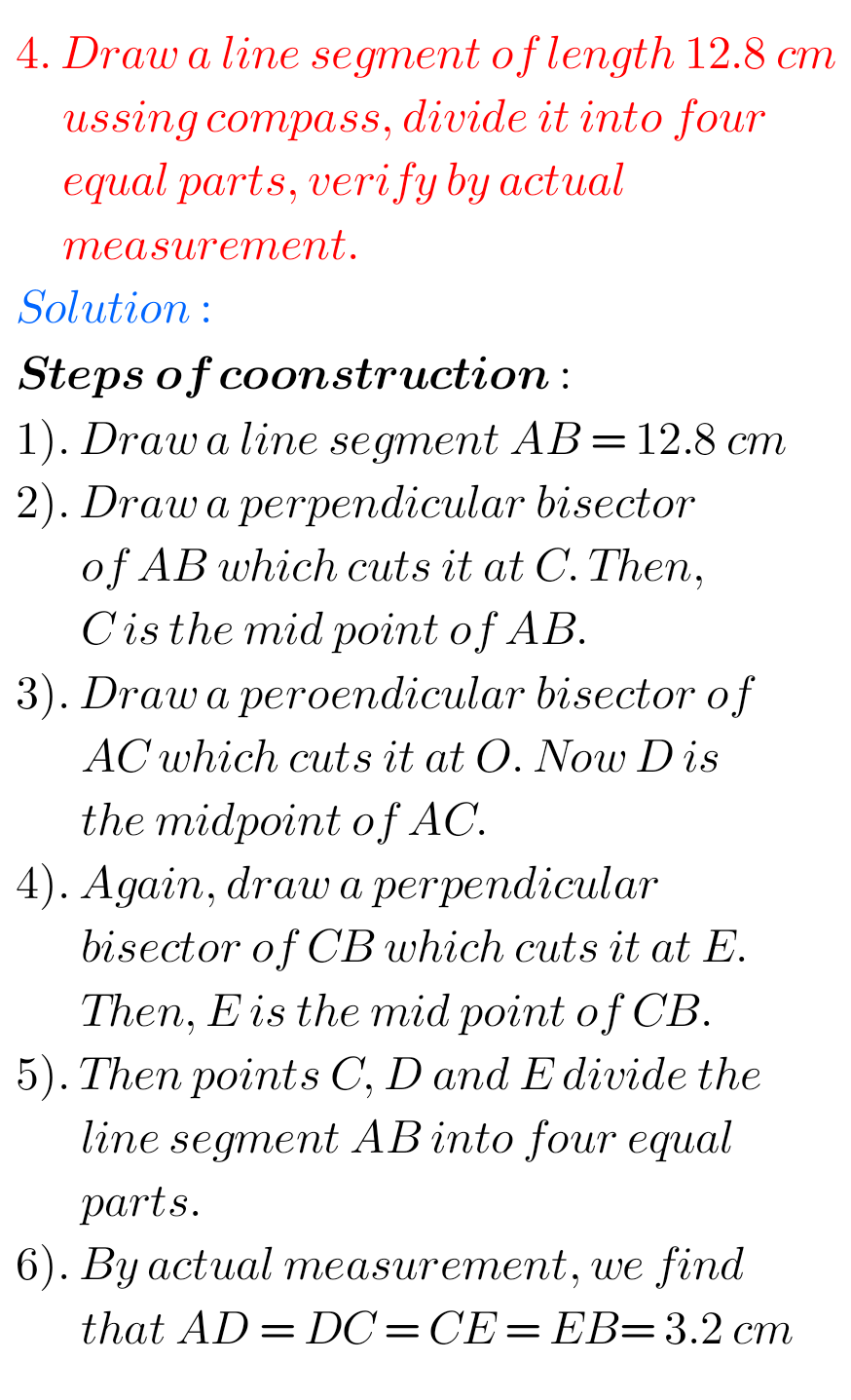## Exercise 14.5 Practical Geometry Solutions Class 6 Maths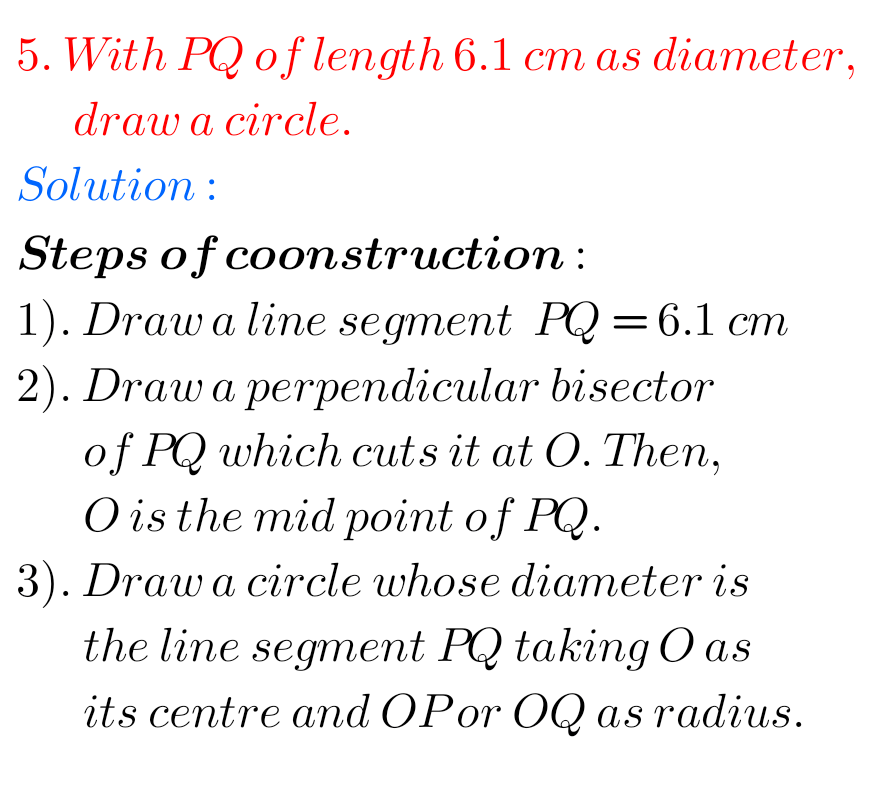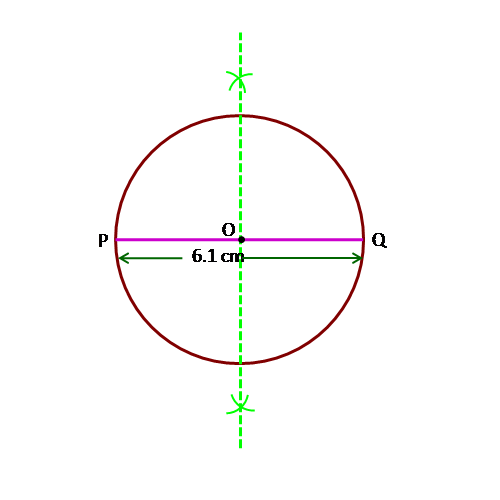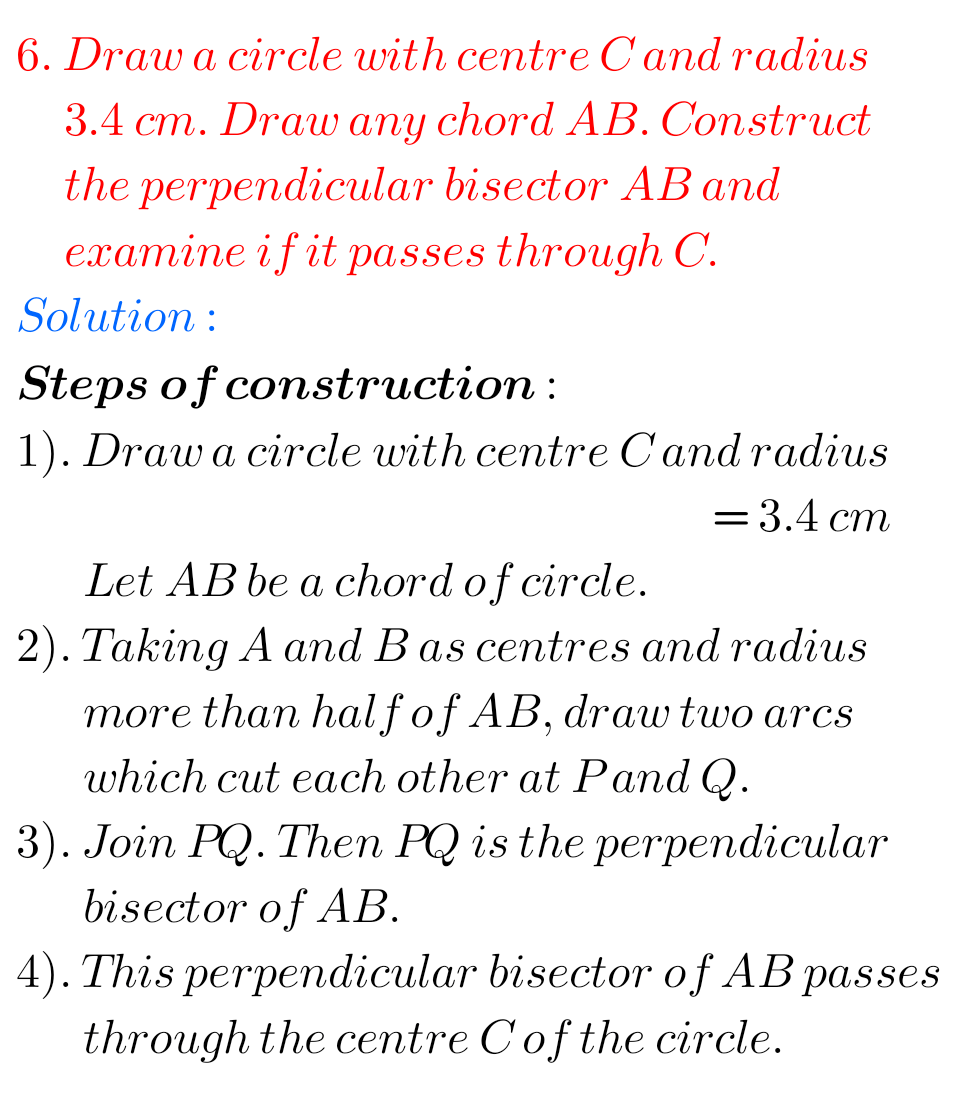## Ncert solutions for Practical Geometry 6th classNote : Observe the solutions and try them in your own method.

Chapter 3 Playing with Numbers

Chapter 4 Basic Geometrical Ideas

Exercise 4.1

Exercise 4.2

Exercise 4.3

Exercise 4.4

Exercise 4.5

Exercise 4.6

Integers

Chapter 6 Integers

Fractions

Chapter 14 Practical Geometry

Exercise 14.1

Exercise 14.2

Exercise 14.3

Exercise 14.4

Exetcise 14.5

Exercise 14.6